# Consider the rectangular cavity (Fig. 2-19) and the mixed-field  formula [Eq. (7-72)1. Choose a trial field where A1 and A2 are  parameters. Determine A1 and Az by the Ritz method, and show that the resultant formula for (or is the exact formula [Eq. (2-95)J. Why do we get an exact solution in this case?

Question
1 views

Consider the rectangular cavity (Fig. 2-19) and the mixed-field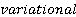formula [Eq. (7-72)1. Choose a trial field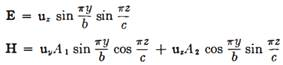where A1 and A2 areparameters. Determine A1 and Az by the Ritz method, and show that the resultant formula for (or is the exact formula [Eq. (2-95)J. Why do we get an exact solution in this case?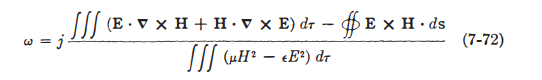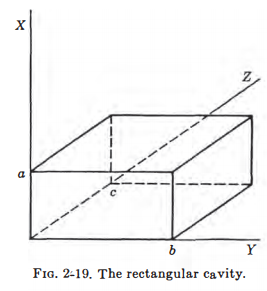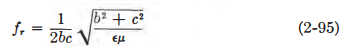### This question hasn't been answered yet.